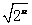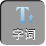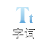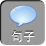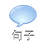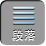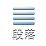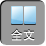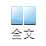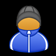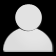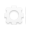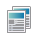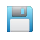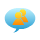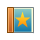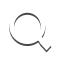-AA+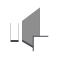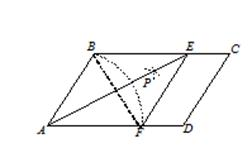∵四边形 ABEF为菱形，∴ BFAE互相垂直平分，∠ BAE＝∠ FAE

OA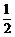AE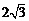．∵菱形 ABEF的周长为 16，∴ AF4

cosOAF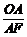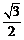．∴∠ OAF30°，∴∠ BAF60°．

∵四边形 ABCD为平行四边形，∴∠ C＝∠ BAD60°．

2、一张矩形纸片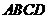，已知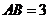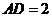，小明按所给图步骤折叠纸片，则线段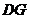长为（ ）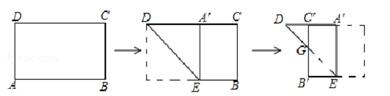A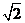B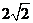C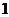D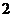1. 如图，将矩形 ABCD沿 GH对折，点 C落在 Q处，点 D落在 AB边上的 E处， EQBC相交于点 F．若 AD8AB6AE4，则△ EBF周长的大小为___________．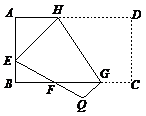RtAEH中，根据勾股定理可得：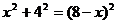，解得 x= 3,即 AH= 3EH= 5

BF=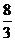, EF=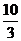.

2、将正方形 ABCD折叠，使顶点 A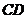边上的一点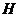重合（不与端点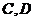重合），折痕交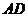于点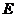，交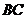于点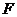，边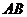折叠后与边交于点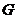，设正方形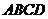的周长为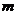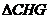的周长为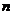，则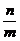的值为（ ）

A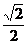B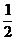C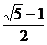D．随点位置的变化而变化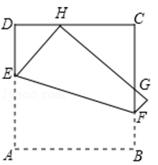CM= xDE= y，则 DM= 2 a-xEM= 2 a-y

∵∠ EMG= 90°，

∴∠ DME+∠ CMG= 90°．

∵∠ DME+∠ DEM= 90°，

∴∠ DEM=∠ CMG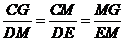,即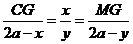CG=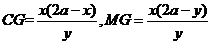CMG的周长为 CM+ CG+ MG=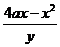RtDEM中， DM 2+ DE 2= EM 2

CM+ MG+ CG=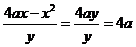= n

3、如图，在矩形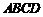中，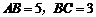,将矩形绕点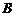按顺时针方向旋转得到矩形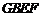，点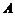落在矩形的边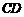上，连接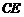，则的长是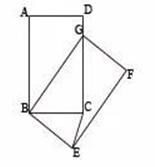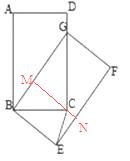1.如图，已知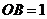，以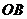为直角边作等腰直角三角形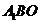再以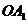为直角边作等腰直角三角形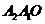，如此下去，则线段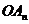的长度为 ．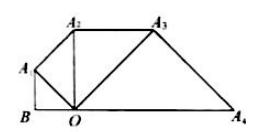AA 1= OA= 1OA 1=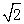OB=∵△ OA 1 A 2为等腰直角三角形，

A 1 A 2= OA 1=OA 2=OA 1= 2

∵△ OA 2 A 3为等腰直角三角形，

A 2 A 3= OA 2= 2OA 3=OA 2= 2∵△ OA 3 A 4为等腰直角三角形，

A 3 A 4= OA 3= 2OA 4=OA 3= 4

∵△ OA 4 A 5为等腰直角三角形，

A 4 A 5= OA 4= 4OA 5=OA 4= 4∵△ OA 5 A 6为等腰直角三角形，

A 5 A 6= OA 5= 4OA 6=OA 5= 8

OA n的长度为